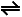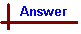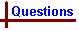Non-ionic equilibrium (General questions) Your feedback on these self-help problems is appreciated. Click here to send an e-mail. Shortcut to Questions Q: 1 2 3 4 5 6 7 8 9 10 11 1 For each of the following reactions write an algebraic expression for the equilibrium constants Kp and Kc and give the relationship between Kp and Kc. (1.) CH4(g) + Cl2(g)CH3Cl(g) + HCl(g) (2.) 2NO(g) + O2(g)2NO2(g) (3.) 4HCl(g) + O2(g)2Cl2(g) + 2H2O(g) (4.) 2Pb(NO3)2(s)2PbO(s) + 4NO2(g) + O2(g) (5.) (NH4)2CO3(s)NH3(g) + NH4HCO3(s) (6.) NH4HCO3(s)NH3(g) + H2O(g) + CO2(g) (7.) CuO(s) + SO3(g)CuSO4(s)2 Give an expression for Kc for each of the following reactions. (1.) 2SO2(g) + O2(g)2SO3(g) (2.) SO2(g) + ½O2(g)SO3(g) (3.) 2SO3(g)2SO2(g) + O2(g) (4.) CaCO3(s)CaO(s) + CO2(g)3 When some nitrogen oxide, oxygen and nitrogen dioxide gases are injected into an evacuated container at a certain temperature the equilibrium 2NO(g) + O2(g)2NO2(g) is established, for which ΔH = -5.66 kJ mol-1. Predict how the following disturbances will affect the concentration of nitrogen dioxide gas in the vessel at equilibrium. (In (1.) to (4.) the volume of the container is fixed.) Write "increase", "decrease" or "no change". (1.) Increasing the temperature (2.) Removing nitrogen(II) oxide gas from the vessel (3.) Adding a catalyst (4.) Adding oxygen gas to the vessel (5.) Compressing the vessel to a smaller volume4 At a suitable temperature the following gas equilibrium is established in a container of fixed volume: N2(g) + O2(g)2NO(g) ΔH for the forward reaction is +180 kJ mol-1. (1.) Find an expression for the equilibrium constant for the reaction. (2.) State the effect upon the equilibrium of each of the following ("L to R", "R to L", or "no effect"): (a) Increased temperature (b) Decreased pressure (c) Increased concentration of oxygen (d) Increased concentration of nitrogen(II) oxide (e) Presence of a catalyst (f) Addition of argon at constant volume5 For the equilibrium H2(g) + I2(g)2HI(g), ΔH = -13 kJ mol-1 at 715K. At 750 K, is the equilibrium constant "greater", "unchanged" or "smaller"?6 When hydrogen iodide (1.000 mole) in a sealed 1.00 litre flask is maintained at a temperature of 225 °C, it decomposes until hydrogen (0.182 mole) is formed. What is the value of Kc for the reaction: 2HI(g)H2(g) + I2(g) at 225 °C ?7 For the equilibrium 2SO2(g) + O2(g)2SO3(g) Kc = 800 M-1 at 525 °C. Calculate the following (at 525 °C): (1.) Kc and Kp for the equilibrium SO2(g) + ½O2(g)SO3(g) (2.) Kp for the equilibrium SO3(g)SO2(g) + ½O2(g)8 In a sealed container, the reaction: CO(g) + Cl2(g)COCl2(g) was allowed to come to equilibrium at a certain temperature. The concentrations of carbon monoxide and carbonyl chloride gases in the mixture were respectively 0.30 M and 0.80 M. If the concentration equilibrium constant is 13.3, find the concentration of chlorine gas in the mixture.9 At 294 K, Kc for the dissociation of dinitrogen tetroxide, N2O4(g)2NO2(g) is 0.48. Calculate the concentration of nitrogen dioxide in equilibrium with 0.36 M dinitrogen tetroxide at 294 K.10 At 600 °C, Kc for the reaction 2SO2(g) + O2(g)2SO3(g) is 4.5. What amount of sulfur trioxide was placed in a 1.0 litre reaction vessel if, at equilibrium at 600 °C, the mixture arising from the above reaction was found to contain oxygen gas (0.200 mole)?11 O2(g) + 2NO(g)2NO2(g) (Kc1) 2NO(g) + Cl2(g)2NOCl(g) (Kc2) NO2(g) + ½Cl2(g)NOCl(g) + ½O2 (Kc3) Express Kc3 in terms of Kc1 and Kc2.Non-ionic equilibrium (General answers) 1 Kp is the equilibrium constant defined in terms of partial pressures, and is related to Kc by the relationship Kp = Kc(RT)Δn gas, where R = 0.0821 L atm K-1 mol-1, T is temperature in K, and Δn gas is the change in no. of moles of gas over the course of reaction (Δn gas = (no. mol. product gases - no. mol recatant gases)). (1.) CH4(g) + Cl2(g)CH3Cl + HCl Kp = pCH3Cl . pHCl / pCH4 . pCl2 Kc = [CH3Cl] [HCl] / [CH4] [Cl2] Kp = Kc. In this example Kp is equal to Kc because there is no change in the no. moles gas from reactants to products. (2.) 2NO(g) + O2(g)2NO2(g) Kp = p2NO2 / p2NO . pO2 Kc = [NO2]2 / [NO]2 [O2] Kp = Kc(RT)-1 (3.) 4HCl(g) + O2(g)2Cl2(g) + 2H2O(g) Kp = p2Cl2 . p2H2O / p4HCl . pO2 Kc = [Cl2]2 [H2O]2 / [HCl]4 [O2] Kp = Kc(RT)-1 (4.) 2Pb(NO3)2(s)2PbO(s) + 4NO2(g) + O2(g) Note that solids and pure liquids do not appear as terms in expressions for K: Kp = p4NO2 . pO2 Kc = [NO2]4 [O2] Kp = Kc(RT)5 (5.) (NH4)2CO3(s)NH3(g) + NH4HCO3(s) Kp = pNH3 Kc = [NH3] Kp = KcRT (6.) NH4HCO3(s)NH3(g) + H2O(g) + CO2(g) Kp = pNH3 . pH2O . pCO2 Kc = [NH3] [H2O] [CO2] Kp = Kc(RT)3 (7.) CuO(s) + SO3(g)CuSO4(s) Kp = 1 / pSO3 = p-1SO3 Kc = [SO3]-1 Kp = Kc(RT)-12 Note that (1.) and (2.) are different equations describing the same reaction, and that the expression for Kc varies according to the way the equation is written. Also note that solids and pure liquids do not appear as terms in expressions for K. (1.) Kc = [SO3]2 / [SO2]2 [O2] (2.) Kc = [SO3] / [SO2] [O2]½ (3.) Kc = [SO2]2 [O2] / [SO3]2 (4.) Kc = [CO2]3 2NO(g) + O2(g)2NO2(g) (ΔH = -5.66 kJ mol-1) (1.) The negative ΔH value indicated the reaction is exothermic. As a result, increasing the temperature will favour the reactants, leading to a decrease in concentration of NO2. (2.) Upon removal of NO the system will re-equilibrate in such a way as to replace the NO just removed. This will lead to a decrease in concentration of NO2. (3.) Adding a catalyst will have no effect on the equilibrium concentration of NO2. Catalysts decrease the time taken to reach equilibrium, but do not affect the equilibrium concentrations once equilibrium is reached. (4.) Upon addition of O2 the system will re-equilibrate in such a way as to use the O2 just added. This will lead to an increase in concentration of NO2. (5.) Since there are fewer moles of gas on the product side (2 mol) than the reactant side (3 mol), increasing the pressure by reducing the volume will move the reaction towards the side which contains fewer moles of gas. This will lead to an increase in concentration of NO2 with increased applied pressure.4 (1.) Kc = [NO]2 / [N2] [O2] (2.) 2NO(g) + O2(g)2NO2(g) (ΔH = +180 kJ mol-1) (a) Since the reaction is endothermic (positive ΔH value), increasing the temperature will make the reaction move from L → R. (b) Since there are equal amounts of gas on each side of the equation, changing the pressure will have no effect on the equilibrium. (c) Increasing the amount of oxygen will make the reaction move from L → R. (d) Increasing the concentration of NO will move the reaction from R → L. (e) The addition of a catalyst will not affect the direction of reaction (ie the equilibrium concentrations will be the same as no catalyst), it will only make the system equilibrate faster. (f) Addition of an inert gas (like argon) at constant volume has no effect on the equilibrium position since the partial pressures of the gaseous components remain the same.5 H2(g) + I2(g)2HI(g) (ΔH = -13 kJ mol-1 at 715K) The equilibrium constant can be expressed in terms of forward and reverse reaction constants: K = kforward / kreverse. Since the forward reaction is exothermic, an increase in temperature will favour the reverse reaction, so kforward will decrease, and kreverse will increase. From the above equation, this will lead to a smaller value of K.6 2HI(g)H2(g) + I2(g) Kc = [H2] [I2] / [HI]2. From the above reaction equation, 1 mole of HI reacting will produce 0.5 mole H2 and 0.5 mole I2. So if 0.182 mol H2 is formed at equlibrium, 0.182 mol I2 must also be present. Since 2 moles HI react to give 1 mole H2, if there are 0.182 mol H2 present, then 2 x 0.182 mol = 0.364 mol HI must have reacted. Therefore the number of moles HI remaining at equilibrium = no. mol HI reactant - no. mol HI consumed = 1.000 mol HI - 0.364 mol HI = 0.636 mol. Substituting these values into the above expression for Kc we obtain Kc = (0.182 mol L-1) x (0.182 mol L-1) / (0.636 mol L-1)2 = 0.0819. Note that in this case Kc is unitless. Referring to the Kc expression it can be seen that units of mol2 L-2 appear on both numerator and demoninator, hence cancelling and leaving the resultant Kc value unitless.7 2SO2(g) + O2(g)2SO3(g) (Kc = 800 L mol-1 at 525 °C) ...Equation 1 (1.) SO2(g) + ½O2(g)SO3(g) ...Equation 2 By considering the expressions of Kc for each of the two equations, the relationship between them can be determined: Kc (Equation 1) = [SO3]2 / [SO2]2 [O2] Kc (Equation 2) = [SO3] / [SO2] [O2]½ It is evident that the expression for Kc (Equation 2) is the square root of the expression for Kc (Equation 1). Therefore the numerical value of Kc (Equation 2) = (800 L mol-1)½ = 28.3 L½ mol -½. This is the basis of the general rule for converting Kc values between equations which differ only by a common factor: If reaction equation with an equilibrium constant Kc is multiplied through by a factor x, the resultant equilibrium constant is (Kc)x. So in this example, where Equation 2 is Equation 1 multiplied through by the factor 0.5, the Kc value for Equation 2 is the square root of Kc for Equation 1. Kp = Kc(RT)Δn gas In this case R = 0.0821 L atm K-1 mol-1, T = (273 + 525) = 798 K, and Δn gas = -0.5. This gives Kp = 28.3 L½ mol -½ x (0.0821 L atm K-1 mol-1 x 798 K) -½ = 3.49 atm -½. Note that the units in this expression cancel to give Kp in atm. From this a general rule can be established - if Δn gas = x, then the units of Kp will be atmx (∴ if Δn gas = 0, Kp will be unitless). (2.) SO3(g)SO2(g) + ½O2(g) ...Equation 3 This is the reverse of Equation 2. We can compare the expressions of Kp for the two equations to determine the relationship between them: Kp (Equation 2) = pSO3 / pSO2 p½O2 Kp (Equation 3) = pSO2 p½O2 / pSO3 This shows that the expression for Kp (Equation 3) is the inverse of the expression for Kp (Equation 2). The Kp value for Equation 3 will therefore be the inverse of the Kp value of Equation 2. ie Kp (Equation 3) = (Kp (Equation 2))-1 = (3.49 atm -½ ) -1 = 0.289 atm½. Note here that the units for Kp are also inverted.8 CO(g) + Cl2(g)COCl2(g) [CO] = 0.30 mol L-1 [COCl2] = 0.80 mol L-1 Kc = 13.3 L mol-1 = [COCl2] / [CO] [Cl2] ∴[Cl2] = [COCl2] / [CO] Kc = 0.80 mol L-1 / 0.30 mol L-1 x 13.3 L mol-1 = 0.20 mol L-19 N2O42NO2 (Kc = 0.48 mol L-1 at 294 K) Kc = [NO2]2 / [N2O4] ∴ [NO2] = (Kc [N2O4])½ = (0.48 mol L-1 x 0.36 mol L-1)½ = 0.42 mol L-1.10 2SO2 + O22SO3 Kc = 4.5 L mol-1 at 600 °C Kc = [SO3]2 / [SO2]2 [O2] [O2] = 0.200 mol L-1 ∴ [SO2] = 0.400 mol L-1 [SO3] = (Kc [SO2]2 [O2])½ = (4.5 L mol-1 x 0.4002 mol2 L-2 x 0.200 mol L-1) = (0.144 mol2 L-2)½ = 0.38 mol L-1 at equilibrium. ∴[SO3] before equilibrium = amount of SO3 present at equilibrium + amount of SO3 converted into SO2 and O2 = 0.38 mol L-1 + 0.400 mol L-1 = 0.78 mol L-1. Volume of reaction vessel = 1L, \ 0.78 mol SO3 was placed into the reaction vessel.11 Kc1 = [NO2]2 / [NO]2 [O2] Kc2 = [NOCl]2 / [Cl2] [NO]2 Kc3 = [NOCl]2 [O2]½ / [NO2] [Cl2]½ Rearranging Kc1 and Kc2: Kc1-½ = [NO] [O2]½ / [NO2] Kc2½ = [NOCl] / [Cl2]½ [NO] Kc1-½ x Kc2½ = [NO] [O2]½ [NOCl] / [Cl2]½ [NO] [NO2] = [O2]½ [NOCl] / [Cl2]½ [NO2] = Kc3.